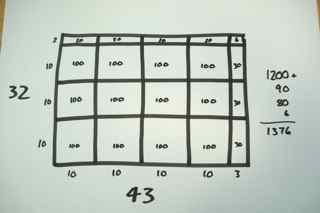## There's more than one way to skin a cat

And there's more than one way to deal with Multiplication. So if your kids are struggling with multi-digit multiplication, try one of these alternatives:

## 1. The Vertical Algorithm

Okay - so here's old faithful. You get the top number and multiply it by the bottom number. Basically it's breaking the 2-digit algorithm into 4 single digit operations, using your knowledge of place value and the distributive law.

The example on the right simplifies this into two steps.

Many kids, and teachers, think that this is the only way to do multi-digit multiplication.

## 2. Draw it as a rectangleWe use multiplication to calculate the area of rectangles. This method can be used to represent the actual multiplication algorithm as well.

In the example, the rectangle drawn is 32 units high and 43 units wide.

Then the rectangles is divided up into simple boxes that are 10 x 10. These are easy to add up - they are equal to 100 each.

The left over bits at the top and sides are smaller rectangles that can be added on.

## 3. Using the diagonal grid - The Lattice Grid

Write the numbers as shown with a diagonal grid or lattice pattern as shown.
As you multiply each number, the result is written in the corresponding triangle.
When you finish, add along the diagonals. The lowest diagonal is units column, the next is the tens column etc.
The link below is a clip from Khan Academy working through an example - worth a look.

## 4. Visual MultiplicationHere's a really visual way to show multiplication.

Represent each multiplicand as a series of parallel diagonal lines.

In the example, 43 is shown as 4 lines and 3 lines sloping up from the left to the right.

The other multiplicand is 32, 3 lines and 2 lines crossing over the first line and going downwards from left ot right.

## 5. Russian MultiplicationMy favourite - love the way this works.

1. Write the two multiplicands beside each other.

2. Halve the number on the left, double the number on the right. If the number on the right ends in a fraction, round it down to the next whole number.

3. Continue until the number on the left gets to 1.

4. Cross out the number on the right if the corresponding number on the left is even.

5. Add the remaining numbers in the right column.

Hope this gives you a few ideas to try out.